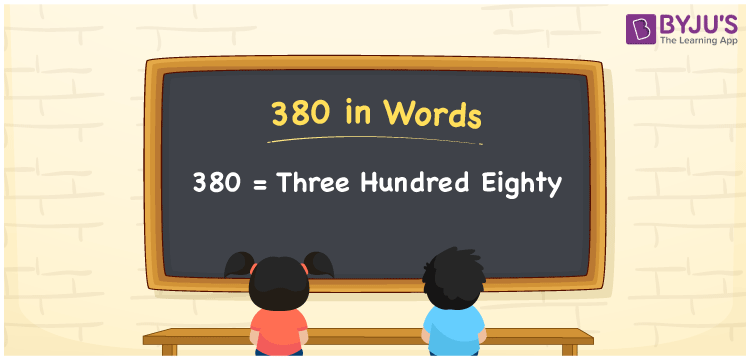# 380 in words

380 in words is written as Three Hundred and Eighty. 380 represents the count or value. The article on Counting Numbers can give you an idea about count or counting. The number 380 is a 3 digit number that is used in expressions related to money, days, distance, length, weight and so on. Let us consider an example for 380. “The first innings score is Three Hundred and Eighty runs”.

 380 in words Three Hundred and Eighty Three Hundred and Eighty in Numbers 380

## 380 in English Words## How to Write 380 in Words?

We can convert 380 to words using a place value chart. The number 380 has 3 digits, so let’s make a chart that shows the place value up to 3 digits.

 Hundreds Tens Ones 3 8 0

Thus, we can write the expanded form as:

3 × Hundred + 8 × Ten + 0 × One

= 3 × 100 + 8 × 10 + 0 × 1

= 380

= Three Hundred and Eighty.

380 is the natural number that is succeeded by 379 and preceded by 381.

380 in words – Three Hundred and Eighty.

Is 380 an odd number? – No.

Is 380 an even number? – Yes.

Is 380 a perfect square number? – No.

Is 380 a perfect cube number? – No.

Is 380 a prime number? – No.

Is 380 a composite number? – Yes.

## Solved Example

1. Write the number 380 in expanded form

Solution: 3 × 100 + 8 × 10 + 0 × 1

We can write 380 = 300 + 80 + 0

= 3 × 100 + 8 × 10 + 0 × 1.

## Frequently Asked Questions on 380 in words

Q1

### How to write the number 380 in words?

380 in words is written as Three Hundred and Eighty.
Q2

### Is 380 divisible by 3?

No. 380 is not divisible by 3.
Q3

### Is 380 a prime number?

No. 380 is not a prime number.